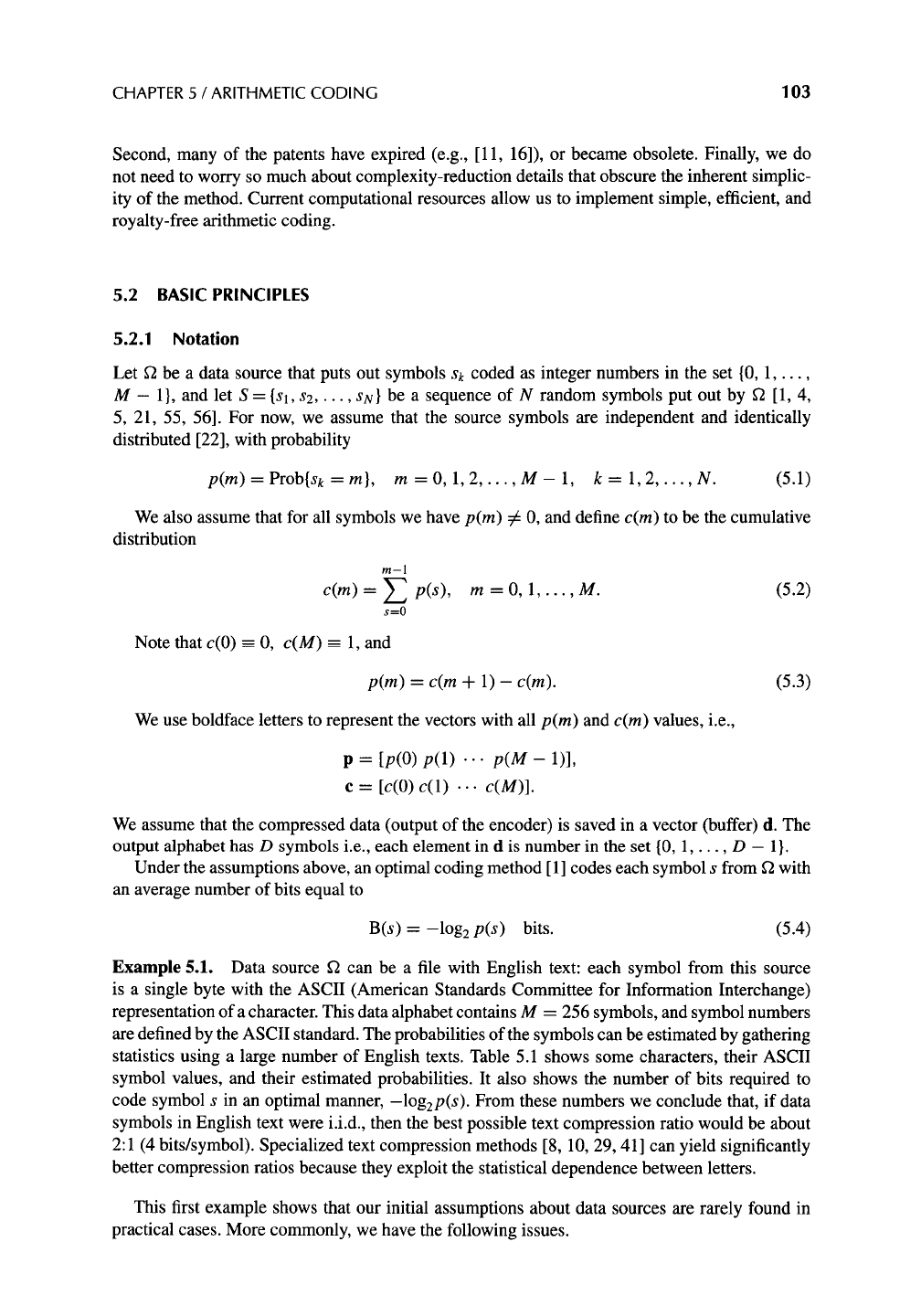CHAPTER 5 / ARITHMETIC CODING 103
Second, many of the patents have expired (e.g., [11, 16]), or became obsolete. Finally, we do
not need to worry so much about complexity-reduction details that obscure the inherent simplic-
ity of the method. Current computational resources allow us to implement simple, efficient, and
royalty-free arithmetic coding.
5.2 BASIC PRINCIPLES
5.2.1 Notation
Let f2 be a data source that puts out symbols sk coded as integer numbers in the set {0, 1 .....
M - 1 }, and let S = {Sl, s2 .....
SN}
be a sequence of N random symbols put out by f2 [1, 4,
5, 21, 55, 56]. For now, we assume that the source symbols are independent and identically
distributed , with probability
p(m)=Prob{sk=m},
m=0,1,2 ..... M-l, k=l,2 ..... N.
(5.1)
We also assume that for all symbols we have
p(m) :7/=
0, and define
c(m)
to be the cumulative
distribution
m-1
c(m) -- Z p(s),
s=0
m = O, 1 ..... M. (5.2)
Note that c(0) -- 0,
c(M)
_--__ 1, and
p(m ) = c(m + 1) - c(m ).
(5.3)
We use boldface letters to represent the vectors with all
p(m)
and
c(m)
values, i.e.,
p = [p(0) p(1) -..
p(M-
1)],
c --- [c(0) c(1) ... c(M)].
We assume that the compressed data (output of the encoder) is saved in a vector (buffer) d. The
output alphabet has D symbols i.e., each element in d is number in the set {0, 1 ..... D -- 1 }.
Under the assumptions above, an optimal coding method [ 1 ] codes each symbol s from f2 with
an average number of bits equal to
B(s) = -log 2
p(s)
bits. (5.4)
Example 5.1. Data source f2 can be a file with English text: each symbol from this source
is a single byte with the ASCII (American Standards Committee for Information Interchange)
representation of a character. This data alphabet contains M = 256 symbols, and symbol numbers
are defined by the ASCII standard. The probabilities of the symbols can be estimated by gathering
statistics using a large number of English texts. Table 5.1 shows some characters, their ASCII
symbol values, and their estimated probabilities. It also shows the number of bits required to
code symbol s in an optimal manner, -log2p(s ). From these numbers we conclude that, if data
symbols in English text were i.i.d., then the best possible text compression ratio would be about
2:1 (4 bits/symbol). Specialized text compression methods [8, 10, 29, 41 ] can yield significantly
better compression ratios because they exploit the statistical dependence between letters.
This first example shows that our initial assumptions about data sources are rarely found in
practical cases. More commonly, we have the following issues.

Get Lossless Compression Handbook now with the O’Reilly learning platform.

O’Reilly members experience live online training, plus books, videos, and digital content from nearly 200 publishers.